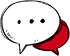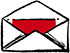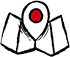# Capstats: fast NIC statistics reporting tool

Submitted by abb on Tue, 09/14/2010 - 18:27

Just came across a nice tool to display NIC statistics, it is called capstats. Capstats is much less CPU intensive that iptraf, so it can be run along with hping3 to monitor its performance.

Example from capstats's website:
>capstats -i nve0 -I 1
1186620936.890567 pkts=12747 kpps=12.6 kbytes=10807 mbps=87.5 nic_pkts=12822 nic_drops=0 u=960 t=11705 i=58 o=24 nonip=0
1186620937.901490 pkts=13558 kpps=13.4 kbytes=11329 mbps=91.8 nic_pkts=13613 nic_drops=0 u=1795 t=24339 i=119 o=52 nonip=0
1186620938.912399 pkts=14771 kpps=14.6 kbytes=13659 mbps=110.7 nic_pkts=14781 nic_drops=0 u=2626 t=38154 i=185 o=111 nonip=0
1186620939.012446 pkts=1332 kpps=13.3 kbytes=1129 mbps=92.6 nic_pkts=1367 nic_drops=0 u=2715 t=39387 i=194 o=112 nonip=0
=== Total
1186620939.012483 pkts=42408 kpps=13.5 kbytes=36925 mbps=96.5 nic_pkts=1 nic_drops=0 u=2715 t=39387 i=194 o=112 nonip=0

So far the tool worked nicely for me, except that on one host I had to run it with -N, otherwise it fails to produce output when there is no traffic on the wire.

## Contacts+32 (0) 2 215 53 58info@gremwell.comGremwell BVBA
Sint-Katherinastraat 24
1742 Ternat
Belgium
VAT: BE 0821.897.133.